C++ Programs to Swap two numbers

In this tutorial you will learn about the C++ Programs to Swap two numbers and its application with practical example.

In this tutorial, we will learn to create a C++ program that will Swap two numbers in C++ programming.

Prerequisites

Before starting with this tutorial we assume that you are best aware of the following C++ programming topics:

• Operators in C++ Programming.
• Basic Input and Output function in C++ Programming.
• Basic C++ programming.

Swapping of Numbers:-

In swapping we swap the data of two variables. In this program, we will use the third variable to swap the data of the first and second variables.

Let us understand with an example.

C++ Programs to Swap two numbers

In every programming language, numbers play a very important role in a programming language. As we all know the c++ is a very powerful language. With the help of the c++ programming language, we can make many programs. We can perform many input-output operations using c++ programming. In today’s tutorial, we take the input numbers from the user. Then we will swap two Numbers with the help of the third variable in the c++ programming language.

With the help of this program, we can Swap two numbers.

Output:-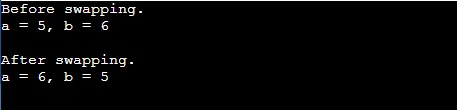In the above program, we have first initialized the required variable.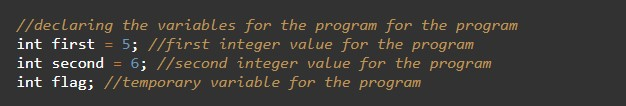• first= it will hold the integer value.
• second = it will hold the integer value.
• flag = it will hold the integer value.

Printing the numbers before the swapping of values.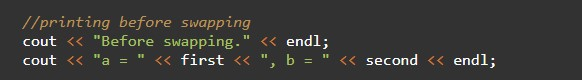Swapping the numbers using a third variable with simple logic.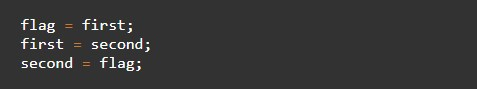Printing the output swapped numbers from the program.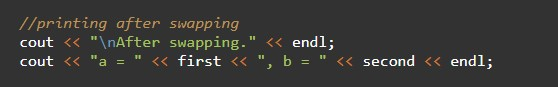In this tutorial we have learn about the C++ Programs to Swap two numbers and its application with practical example. I hope you will like this tutorial.